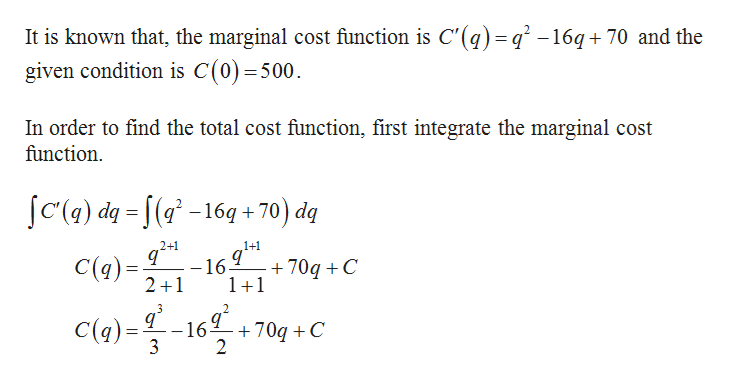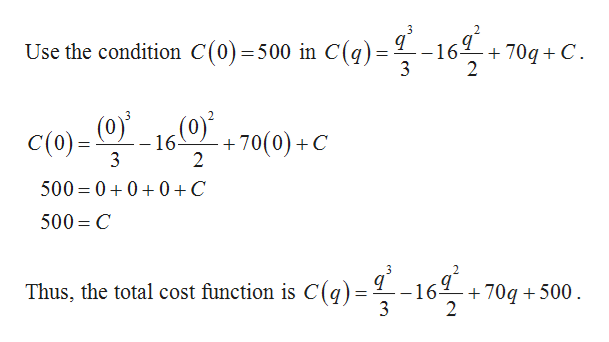# 1. The marginal cost function for a company is given by C'(q)=q^2-16q+70 dollars/unit, where q is the quantity produced. If C(0)=500, find the total cost of producing 20 units.

Question
125 views
1. The marginal cost function for a company is given by C'(q)=q^2-16q+70 dollars/unit, where q is the quantity produced. If C(0)=500, find the total cost of producing 20 units.
check_circle

Step 1help_outlineImage TranscriptioncloseIt is known that, the marginal cost function is C'(q) = q' -16q+ 70 and the given condition is C(0)=500 In order to find the total cost function, first integrate the marginal cost function =S(a? -16q +70) dq 2+1 1+1 q C(a) 2 1 +70qC 1+1 -16 - C(q)=16g -+70q + C 2 fullscreen
Step 2help_outlineImage Transcriptionclose3 -16 Use the condition C(0) =500 in C(q)= 3 704C 2 (0 16(0 -70(0)+C 2 C(0)= 3 500 00 0 +C 500 C 3 Thus, the total cost function is C(q)= L-16+ +70q500 fullscreen

### Want to see the full answer?

See Solution

#### Want to see this answer and more?

Solutions are written by subject experts who are available 24/7. Questions are typically answered within 1 hour.*

See Solution
*Response times may vary by subject and question.
Tagged in
MathCalculus

### Other